Home » 1 to 8 Demultiplexer PLC ladder diagram
PLC Tutorials

# 1 to 8 Demultiplexer PLC ladder diagram

## 1 to 8 Demultiplexer PLC

This is PLC Program to implement 1:8 De-multiplexer.

## Problem Description

Implement 1:8 Demultiplexer in PLC using ladder diagram programming language.

### Problem Diagram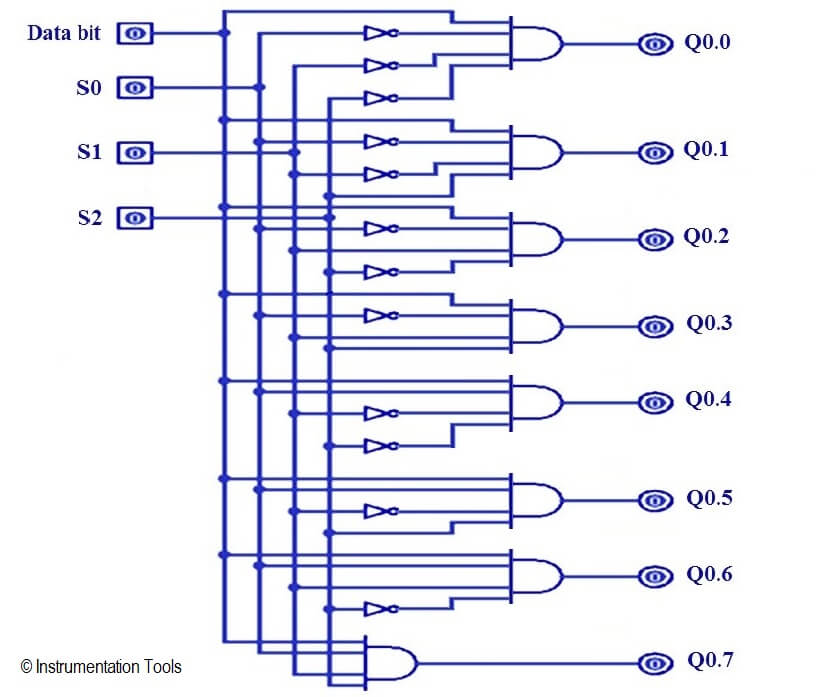### Problem Solution

• Demultiplexer has one data input Di and three select inputs S0, S1 and S3 and 8 outputs Q0.0 to Q0.7.
• To select “n” outputs, we need m select lines such that 2^m = n. Depending on the output. The selection of one of the n outputs is done by the select pins.
• Realize the de-multiplexer using Logic Gates. Here we will configure de-multiplexer using ladder language.
• When three switches are OFF and Di input is pressed then first output will be ON.As per table we can activate output by switching combination.

### 1:8 DeMultiplexer Truth Table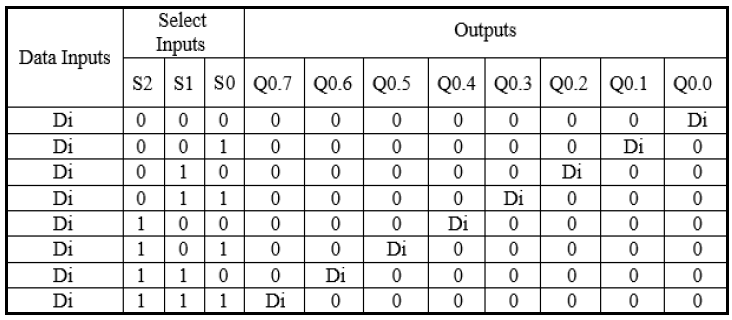### List of inputs/outputs

• S0 :- I0.0
• S1 :- I0.1
• S3 :- I1.0

#### List of outputs

• Output1 :- Q0.0
• Output 2 :- Q0.1
• Output 3 :- Q0.2
• Output 4 :- Q0.3
• Output 5 :- Q0.4
• Output 6 :- Q0.5
• Output 7 :- Q0.6
• Output 8 :- Q0.7

## Ladder diagram for 1 : 8 Demultiplexer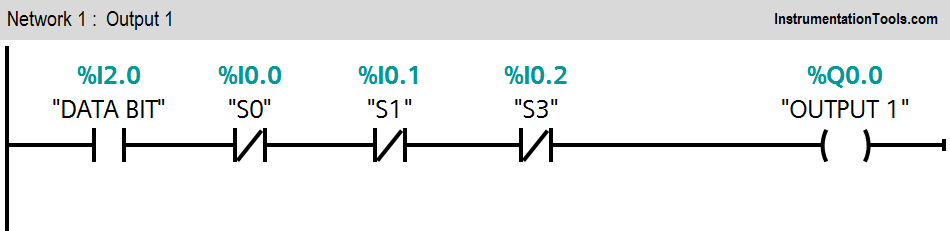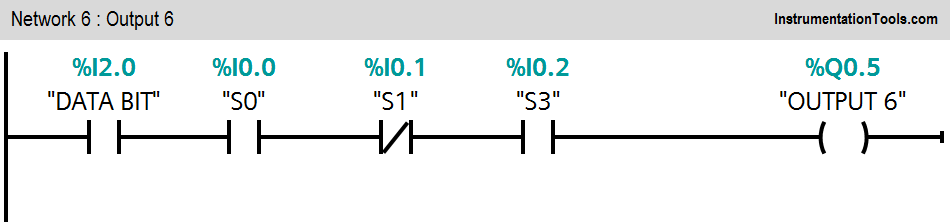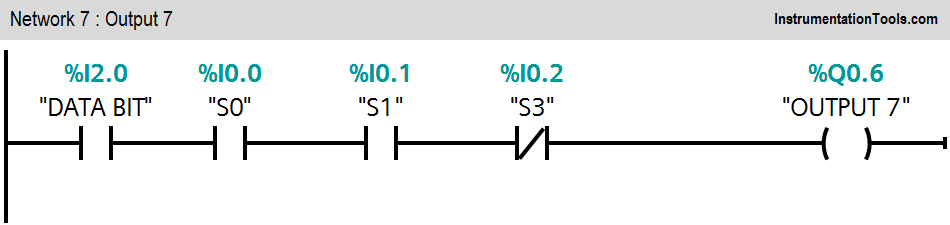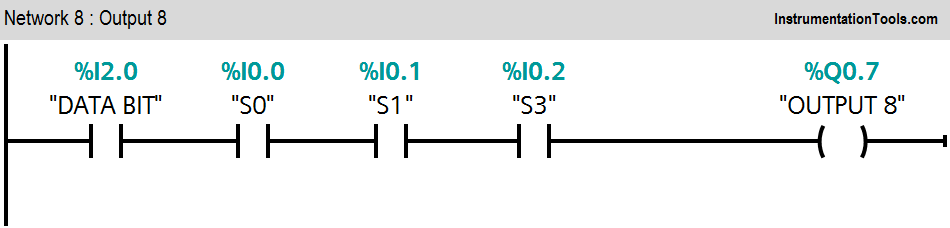### Program Description

For this application we used S7-1200 PLC and TIA portal software for programming.

#### Network 1 :-

If Data bit (I2.0) is ON and all inputs are OFF (I0.0=0, I0.1=0 and I0.2=0), Output 1 (Q0.0) will be ON.

#### Network 2 :-

If data bit (I2.0) is ON, input 1 (I0.0) OFF, input 2 (I0.1) OFF and input 3 (I0.2) is ON, Output 2 (Q0.1) will be ON.

#### Network 3 :-

If data bit (I2.0) is ON, input 1 (I0.0) OFF, input 3 (I0.2) OFF and input 2 (I0.1) is ON, Output 3 (Q0.2) will be ON.

#### Network 4 :-

If data bit (I2.0) is ON, input 2 (I0.1) ON, input 3 (I0.2) ON and input 1 (I0.0) is OFF, Output 4 (Q0.3) will be ON.

#### Network 5 :-

If input 2 (I0.1) and input 3 (I0.2) are OFF and input 1 (I0.0) is ON, Output 5 (Q0.4) will be ON.

#### Network 6 :-

If data bit (I2.0) is ON, input 1 (I0.0), input 3 (I0.2) ON and input 2 (I0.1) is OFF, Output 6 (Q0.5) will be ON.

#### Network 7 :-

If data bit (I2.0) is ON, input 1 (I0.0), input 2 (I0.1) ON and input 3 (I0.2) is OFF, Output 7 (Q0.6) will be ON.

#### Network 8 :-

If data bit (I2.0) is ON, input 1 (I0.0), input 2 (I0.1) and input 3 (I0.2) ON, Output 8 (Q0.7) will be ON.

Note :- Above application may be different from actual application. This example is only for explanation purpose only. We can implement this logic in other PLC also. This is the simple concept of 1:8 Demultiplexer, we can use this concept in other examples also.

All parameters considered in example are for explanation purpose only, parameters may be different in actual applications. Also all interlocks are not considered in the application.

#### Animation of Electromagnetic Relay

This website uses cookies to improve your experience. We'll assume you're ok with this, but you can opt-out if you wish. Accept Read More

WordPress Image Lightbox

Send this to a friend